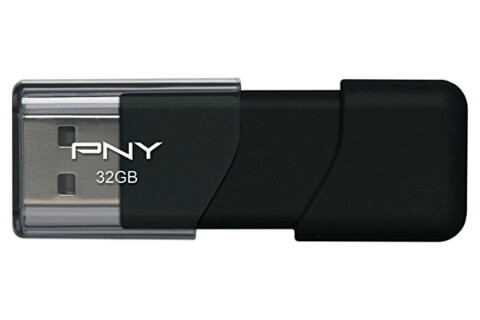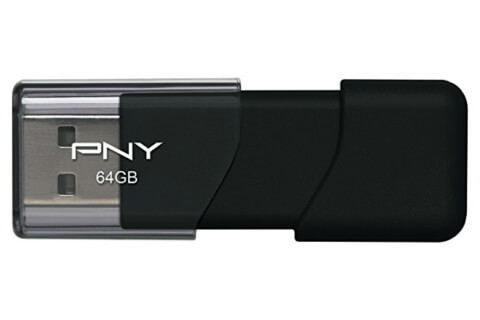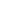Share

# What does "bits per die" mean?

Answer: "Bits per die" is a phrase that refers to the storage density of flash memory. The "die" is the physical block of memory and "bits" refers to the number of bits (how much data) can be stored in that die.

Bits per die is not a very technical measurement, because the size of a die can change. So the storage density of a small die would be greater than a larger die if they both stored the same number of bits. Similarly, if two dies are the same size (such as a keychain flash drive), the bits per die must increase to provide more storage.

For example, the 32GB keychain flash drive below can store 32,000,000,000 bytes, which is equal to 256,000,000,000 bits per die.The 64GB flash drive below has twice the density, or 512,000,000,000 bits per die.The actual storage density of a flash storage device can be determined using the following calculation:

Storage density = the total number of bits in a storage device ÷ the size of the die.

#### Published: March 29, 2018 — by Per Christensson

Answer from the PC Help Center
https://pc.net/helpcenter/what_is_bits_per_die